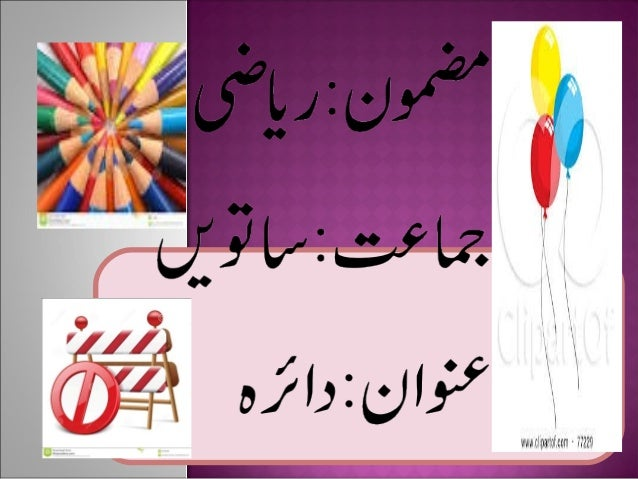Successfully reported this slideshow.Upcoming SlideShare
×

# Circle urdu

390 views

Published on

The power point explains the concepts of major and minor arcs,circular regions,angles in circle,half circle and the types of angles in a circle.

Published in: Education
• Full Name
Comment goes here.

Are you sure you want to Yes No• Be the first to comment

• Be the first to like this

### Circle urdu

1. 1. O
2. 2. O A
3. 3. O P Q A
4. 4. O R S
5. 5. . . . . . . . J A P S K T B
6. 6. . . P . . Q . R A C .B
7. 7. .Y A .X B AB =
8. 8. .Y A .X B
9. 9. .Y A .X B
10. 10. A P Q B Therefore, m∟APB = m∟AQB
11. 11. P A B O Q Therefore, m∟PBQ = 9 0 0 m∟PAQ = 900
12. 12. S P O R Q